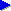Home# Binary representation in computing and Java

On the previous page, we introduced the notion of binary representation of numbers. In binary, numbers are represented in terms of only two digits, 0 and 1, or "off" and "on". This is a convenient representation for computer circuitry and storage media.

Apart from the number system itself, there are some important differences between how we as humans generally use numbers and how binary numbers are used in practice in computing. In everyday usage, we don't usually express numbers as a fixed number of digits unless it's really convenient for some reason (for example, in some post code systems). We just use as many digits as necessary, and in principle numbers can be of arbitrary length.

For computers, letting numbers be "any old length" isn't generally very convenient. It's usually more convenient to define the precise length (in number of binary digits) of the numbers that the computer manipulates or stores.1 In practice, there isn't just one single digit length, but rather several related lengths.

On the next page, we look at the common binary number lengths used in computing.

1. This necessity for a fixed number of digits happens on various levels. For example, if we have a file containing a list of numbers, it's much easier to find the nth number in the file if each number has some fixed number of digits. Similarly, it's only really practical to design memory where each "position" in memory can store some fixed number of binary digits. And to design the circuitry that actually manipulates numbers (performs additions etc), an tiny bit of extra circuitry needs to be added for each extra digit that the computer is to be capable of processing: in other words, we have to specify a fixed number of digits as the size of the numbers that the computer can "natively" manipulate.